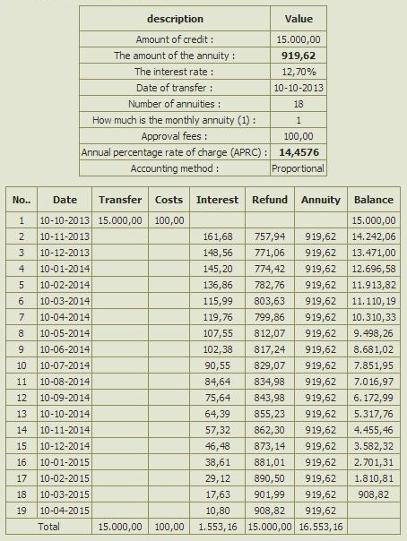# Calculation annuity

## Calculation annuity and amortization schedule### Proportional or simple methodProportional interest expense is ordinary. If we have an annual rate of 10% (10% of which are realized in 365 days), implies that it is in a single day 1/365 of the 10%, 10 days 10/365 10% ..., that is proportional to share.Conformal or compound method.In the conformal mode, the previously accrued interest is included in the basis for calculation, so the interest is increasing over the period. This method is also called interest rate method. Loans with conformal mode of calculation are slightly cheaper.Amortization scheduleAmortization schedule shows us how to move our debt. You can prepare it with annuity payment calculator. Since at the beginning of the period of our long high, at the beginning of pay much interest. These are both of reduced down also reduced. It should be noted that when the pay back half of the meal, we have not yet paid off half the debt. What is the balance of the liability can be determined by an amortization schedule.

For both the calculation formula are quite complex. Let's meet for the first proportional manner. I copied this formula with Excel so that it will be useful to you as well.

### = D6*((((1+(E6/(1200/E8)))^F6)*((1+(E6/(1200/E8)))-1))/(((1+(E6/(1200/E8)))^F6)-1))And a formula similar way:=D6*(1/((((1+(100*(((1+E6/100)^(E8/12))-1))/100)^F6)-1)/(((1+(100*(((1+E6/100)^(E8/12))-1))/100)^F6)*((1+(100*(((1+E6/100)^(E8/12))-1))/100)-1))))Description of the contents of cells:D6 - the principal loanE6 - the annual interest rateF6 - the number of installmentsE8 - how much is the monthly annuity

Make a Free Website with Yola.